## 1. Introduction

In this tutorial, we’ll explain how to find common elements in two sorted arrays.

## 2. Common Elements of Two Sorted Arrays

In this problem, we have two sorted arrays:and. Our task is to find the common elements.

For instance, ifand, our algorithm should outputas the result.

To find the common elements efficiently, it should use the fact thatandare already sorted. Additionally, we’ll also require the output array to be non-descending as the input arrays.

We’ll also assume thatandcan contain duplicates. So, if a common element is repeated twice in, and thrice in, we’ll include it twice in the result array. For instance:## 3. Finding Common Elements in Linear Time

Let’s start with the naive algorithm that doesn’t use the fact thatandare sorted:For each, the algorithm iterates over the entireto check if. So, it has antime complexity, which impliesifandare comparable. What can we change in this algorithm to get common elements faster?

Well, since the arrays are sorted, there’s no point in iterating overif. Each of those is greater than, so we can conclude that. The converse is also true: if, we can discard.

That way, we iterate over each array only once. So, we don’t need nested loops. Instead, we can start withand. When, we discardby incrementing. Similar goes forif. If, we appendto the result array and update both counters. The loop stops once we reach the end ofor. This approach is similar to the merge step in the Merge Sort algorithm:As a result, the algorithm’s time complexity is linear:.

### 3.2. Example

Let’s use the above example to show how the algorithm works.

Initially,is empty, and bothandpoint to the first elements: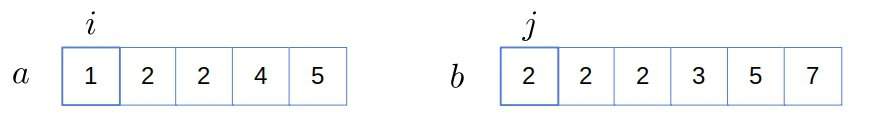Since, we increment: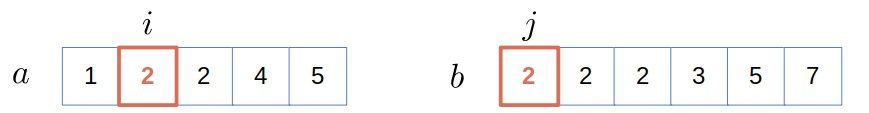Now, we have a match, so we append 2 toand increment both counters: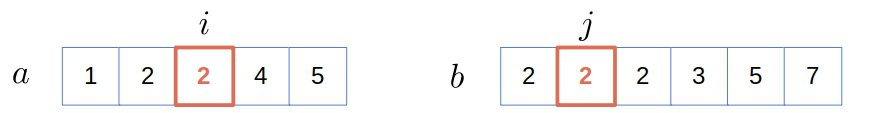We have a match again, so we do the same as in the previous step: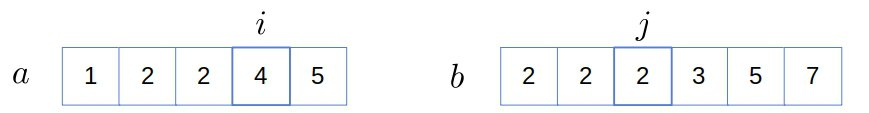Now,, so we discardand move on to: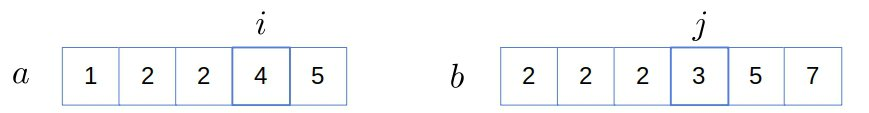The situation is the same (), so we increment only: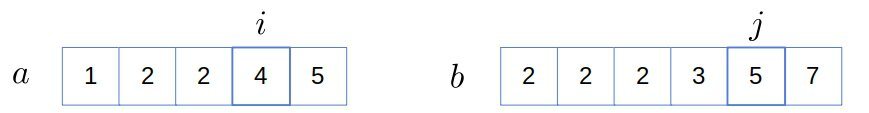Since, we increment only: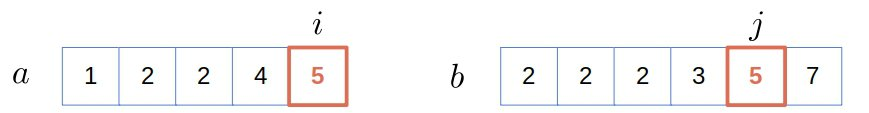We found a new common element, so we add it toand increment both counters: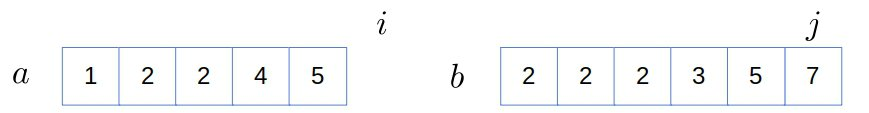Finally,, so we stop and output the result:.

## 4. Finding Common Elements in Logarithmic Time

Let’s suppose that. Then, for each, we can look forinwith the logarithmic binary search. Since, if the binary search findsat the-th position in, we can discard all the element to the left and search forin the remainder:.

Similarly, let’s say the binary search doesn’t findin, but the last index it checks inis. If, we search forin. On the other hand, if, it can still happen that, so we don’t discard.

### 4.1. Pseudocode

Here’s how the above algorithm works:The worst-case time complexity is. If, we can considerto be a constant with respect to. In that case, the algorithm has an approximatecomplexity. However, if, we get analgorithm. That’s still better than the naive approach but worse than theruntime of the two-side linear search.

## 5. Conclusion

In this article, we presented two efficient ways of finding common elements of two sorted arrays. One approach has ancomplexity, whereas the other combines binary and linear searches and runs in antime.